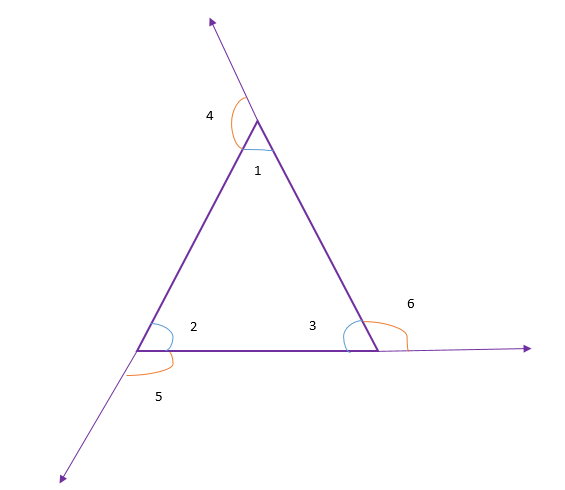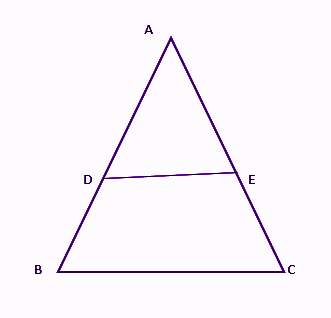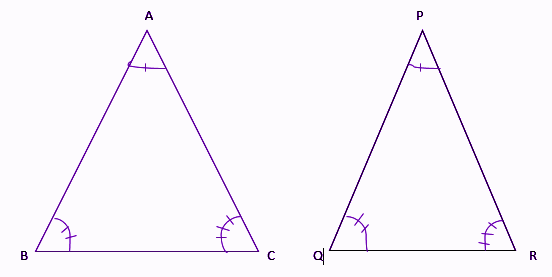# Triangle Theorems

Triangle theorems are basically stated based on their angles and sides. Triangles are the polygons which have three sides and three angles. Now, if we consider the sides of the triangle, we need to observe the length of the sides, if they are equal to each other or not. If there are no sides equal then it is a scalene triangle. If there exist any two sides equal to a triangle, then it is an isosceles triangle. If all the sides are equal in length, then such triangles are called an equilateral triangle.

Now, if we consider the triangles based on the interior angles, they are again classified into three types. If all the angles are less than 90 degrees, then the triangle is called an acute angle triangle. If anyone of the angles is at 90 degrees, then the triangle is known as a right-angled triangle. If measure of any one of the angles is more than 90 degrees, then it is said to be an obtuse angle triangle.

Since we have understood the different types of triangles, let us see the theorems based on triangles here.

## List of Triangle Theorems

Though there are many theorems based on triangles, let us see here some basic but important ones.

Theorem 1: The sum of all the three interior angles of a triangle is 180 degrees.

Suppose ABC is a triangle, then as per this theorem;

∠A + ∠B + ∠C = 180°

Theorem 2: The base angles of an isosceles triangle are congruent.

Or

The angles opposite to equal sides of an isosceles triangle are also equal in measure.

Suppose a triangle ABC is an isosceles triangle, such that;

AB = AC [Two sides of the triangle are equal]

Hence, as per the theorem 2;

∠B = ∠C

Where ∠B and ∠C are the base angles.

Theorem 3: The measure of the exterior angle of a triangle is equal to the sum of the corresponding interior angles.

For a triangle, ABC, ∠1, ∠2 and ∠3 are interior angles. And ∠4, ∠5 and ∠6 are the three exterior angles.Now as per the theorem 3;

∠4 = ∠2 + ∠3

∠5 = ∠1 + ∠3

∠6 = ∠1 + ∠2

## Triangles Theorems For Class 10

Previously we learned about the basic triangle theorems. Now here we will learn about the theorems which are covered for Class 10 syllabus. One of the key theorems explained majorly for trigonometry is Pythagoras theorem.

Theorem 1: If a line is drawn parallel to one side of a triangle to intersect the midpoints of the other two sides, then the two sides are divided in the same ratio.

Suppose ABC is a triangle and DE is a line parallel to BC such that it intersects AB at D and AC at E.Hence, as per the theorem:

Theorem 2: If a line divides any two sides of a triangle in the same ratio, then the line is parallel to the third side.

Suppose ABC is a triangle and a line DE divides the two sides of triangle AB and AC in the same ratio, such that;

Theorem 3: If in two triangles, corresponding angles are equal, then their corresponding sides are in the same ratio and hence the two triangles are similar.

Let ABC and PQR are two triangles.Then as per the theorem,

AB/PQ = BC/QR = AC/PR (If ∠A = ∠P, ∠B = ∠Q and ∠C = ∠R)

And ABC ~ ∆PQR

Theorem 4: If in two triangles, the sides of one triangle are proportional to the sides of the other triangle, then their corresponding angles are equal and hence the two triangles are similar.

Let ABC and PQR are two triangles, then as per the theorem;

∠A = ∠P, ∠B = ∠Q and ∠C = ∠R (if AB/PQ = BC/QR = AC/PR)

And ABC ~ PQR